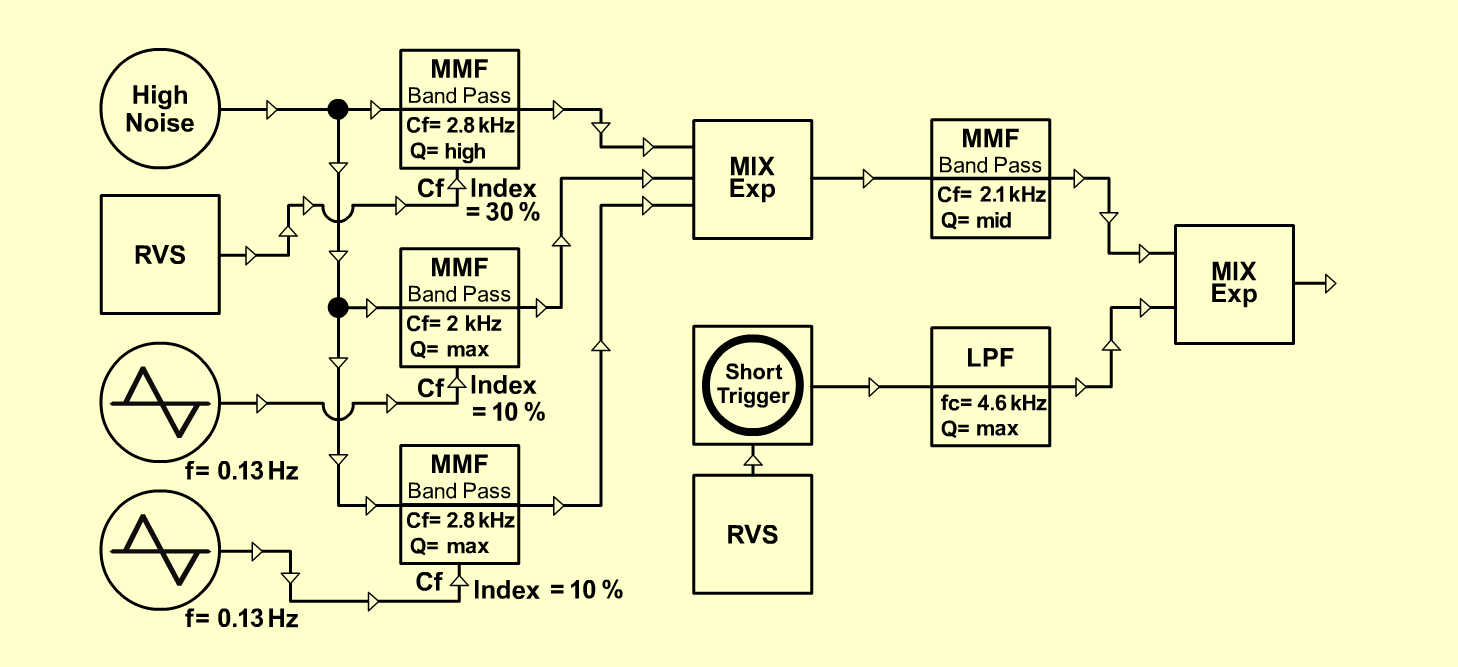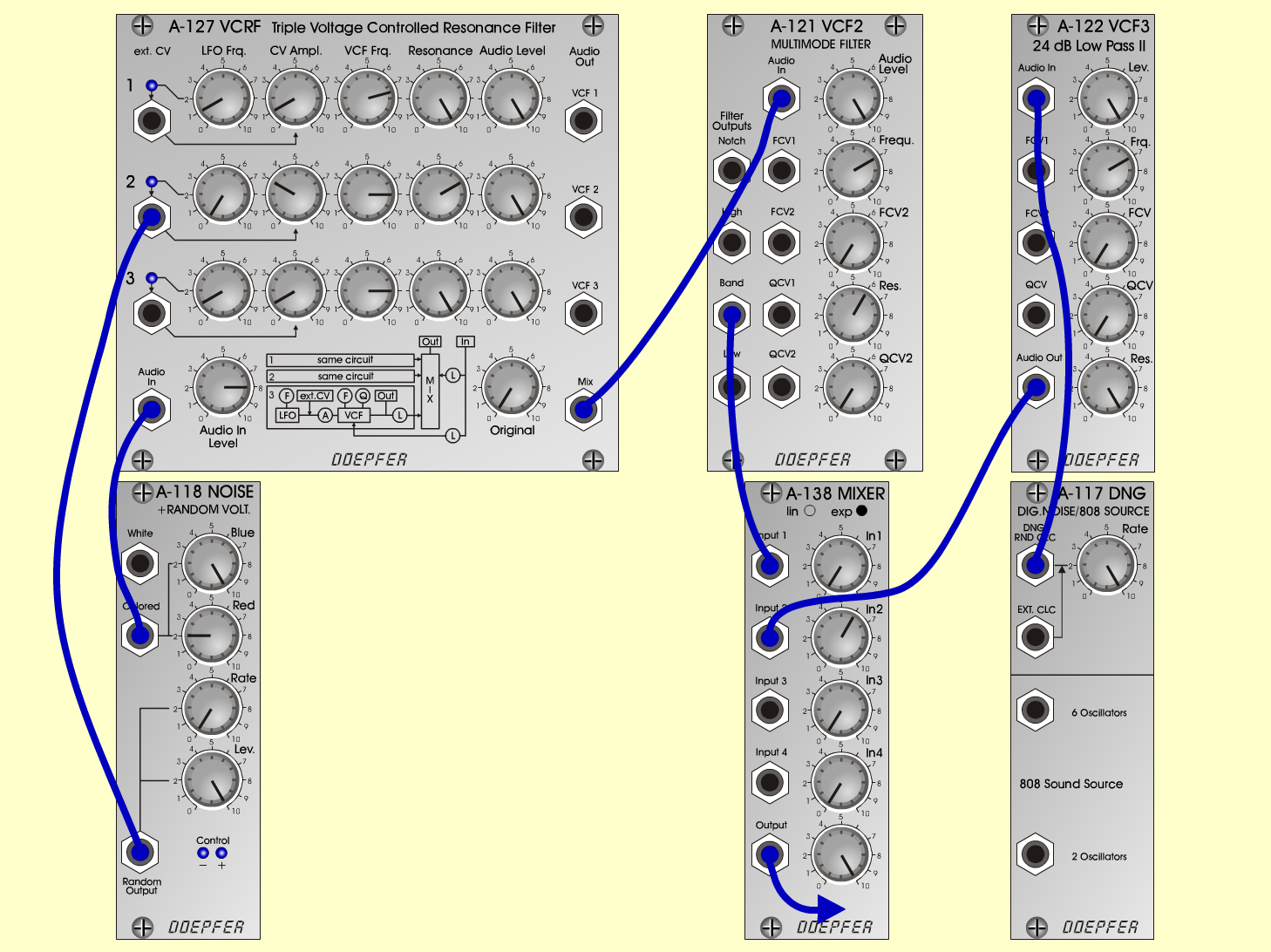Cosmic RaysExperimentalD o e p f e r A - 100 Connections: Settings: A-118 (Colored)  <=>  A-127 (Audio In) A-118 (Random)  <=>  A-127 (ext. CV 2) A-127 (Mix)  <=>  A-121 (Audio In) A-121 (Band)  <=>  A-138exp (Input 1) A-117 (DNG)  <=>  A-122 (Audio In) A-122 (Audio Out)  <=>  A-138exp (Input 2) A-138exp (Output)  <=> Amplifier A-117 (Rate = 10) A-118 (Blue = 10, Red = 2, Rate = 0, Level = 10) A-121 (Audio Level = 10, Freq = 7, Res = 6) A-122 (Level = 10, Freq = 7, Res = 10) A-127 (Audio In = 8, Original = 0; Row 1: LFO Freq = 1, CV Ampl = 1, VCF Frq = 7.5, Res = 10, Audio Level = 10; Row 2: CV Ampl = 3, VCF Frq = 8, Res = 7, Audio Level = 10; Row 3: LFO Freq = 1, CV Ampl = 1, VCF Frq = 8, Res = 10, Audio Level = 10) A-138 (In 1 = 10, In 2 = 6, Out = 10) Josef MuellerSound sample Cosmic Rays